# pheatmap: create annotated heatmaps in R (detailed guide)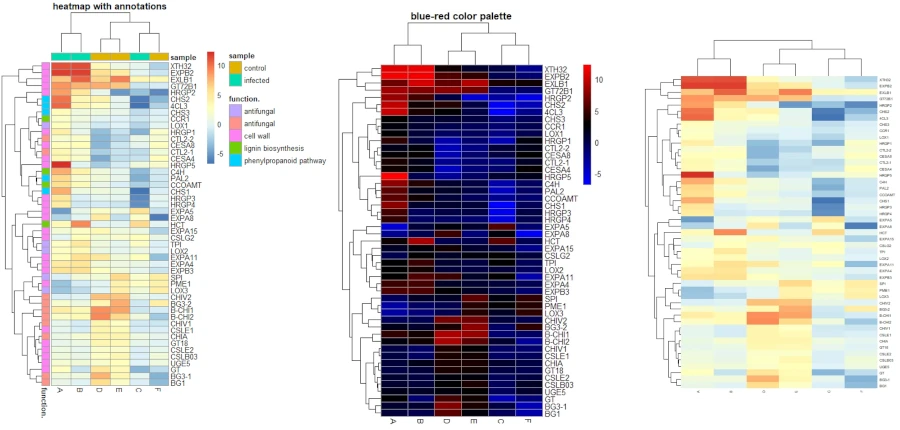### Page content

In bioinformatics, heatmaps are commonly used to visualize gene expression changes across multiple genes and conditions. This article describes how to create clustered and annotated heatmaps for visualization of gene expression data obtained from RNA-seq experiments using a pheatmap R package.

## Install pheatmap

If you have not installed pheatmap package, you can install it using `install.packages()` function.

``````# install pheatmap
install.packages("pheatmap")
``````

You need to load the pheatmap library for creating the heatmaps. I am using the RStudio console with R version 4.2.1

``````# load pheatmap (v1.0.12)
library(pheatmap)
``````

For this pheatmap tuotrial, I will use the gene expressiion dataset generated from RNA-seq experiment in cotton plant in response to pathogenic infection. For simplicity of understanding, I have put the conditions names as A to F. You can import the CSV dataset using `read.csv()` base R function. You can read my article on different ways to import CSV datasets in R.

``````# load dataset
# convert to matrix
df_mat = data.matrix(df)
# view first 5 genes of data
# output
A         B         C        D        E         F
B-CHI1 4.505700  3.260360 -1.249400  8.89807  8.05955 -0.842803
CTL2-1 3.508560  1.660790 -1.856680 -2.57336 -1.37370  1.196000
B-CHI2 2.160030  3.146520  0.982809  9.02430  6.05832 -2.967420
CTL2-2 1.884750  2.295690  0.408891 -3.91404 -2.28049  1.628820
CHIV1  0.255193 -0.761204 -1.022350  3.65059  2.46525 -1.188140
``````

Tip: While importing a dataset, you should always make gene names columns as row names. Otherwise, you would encounter an error while creating a heatmap with pheatmap.

## Create heatmap

### Basic heatmap

You can create a basic clustered heatmap using the pheatmap. You need to pass the data matrix to the `pheatmap()` function.

``````pheatmap(df_mat, main = "basic heatmap")
``````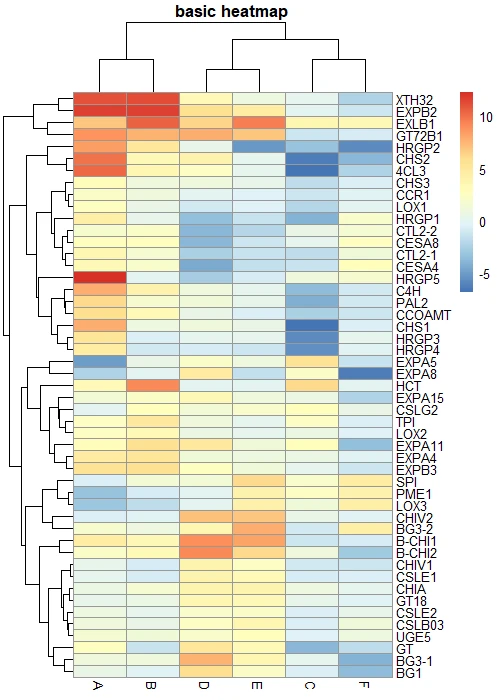### Scale heatmap

pheatmap provides a parameter `scale` to rescale the default values in column or row direction. The rescaling is useful when the values in the dataset are very different from each other. For example, some gene expression values are very high and some are very low.

``````# row scaling
pheatmap(df_mat, scale = "row", main = "row scaling")

# column scaling
pheatmap(df_mat, scale = "column", main = "column scaling")
``````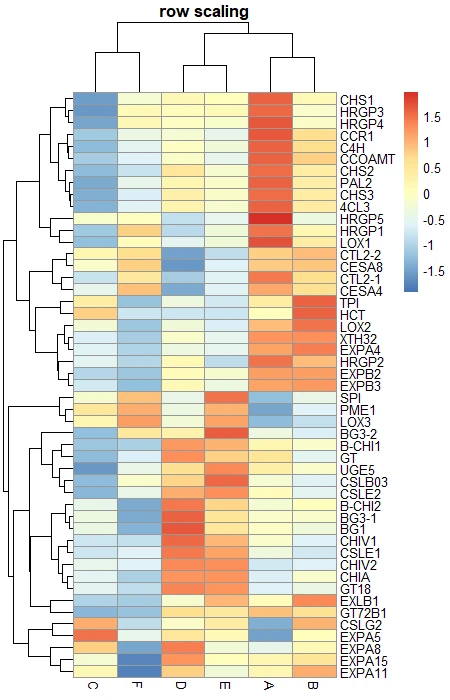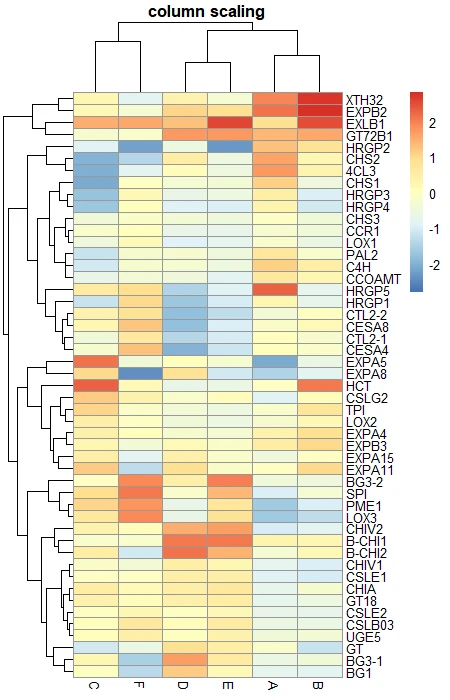### Row and column clustering

By default, pheatmap applies hierarchical clustering to both rows and columns. You can choose if you want to display row/columns clustering or not by using `cluster_rows ` and `cluster_cols` options.

``````# No row clustering
pheatmap(df_mat, cluster_rows = FALSE, main = "no row clustering")

# No column clustering
pheatmap(df_mat, cluster_cols = FALSE, main = "no column clustering")
``````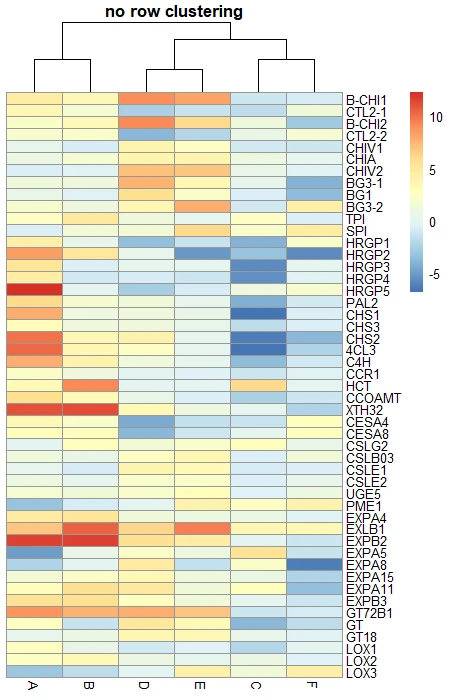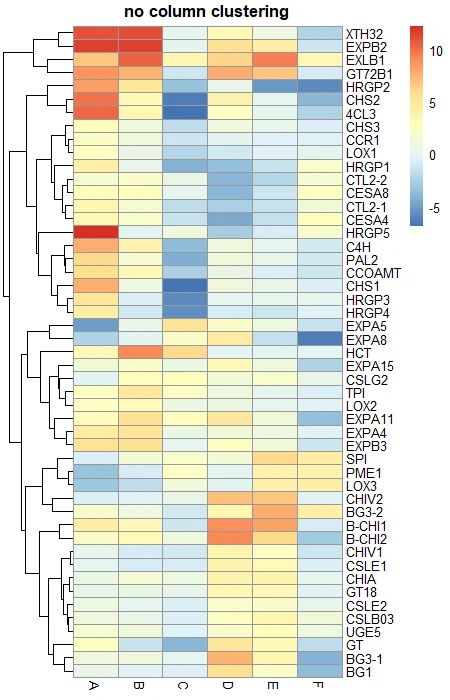### Color palette

You can change the color palette of the heatmap using the `colorRampPalette()` function, which returns a fixed number of colors.

``````# green and red color palette
color <- colorRampPalette((c("red", "black", "green")))(50)
pheatmap(df_mat, color = color, main = "red-green color palette")

# blue and green color palette
color <- colorRampPalette((c("blue", "black", "red")))(50)
pheatmap(df_mat, color = color, main = "blue-red color palette")
``````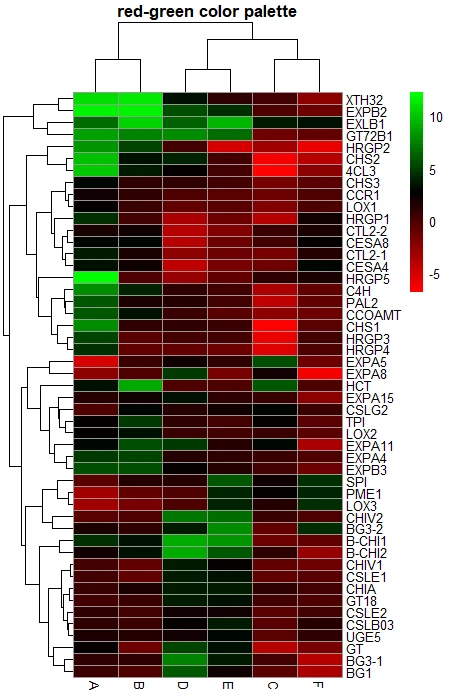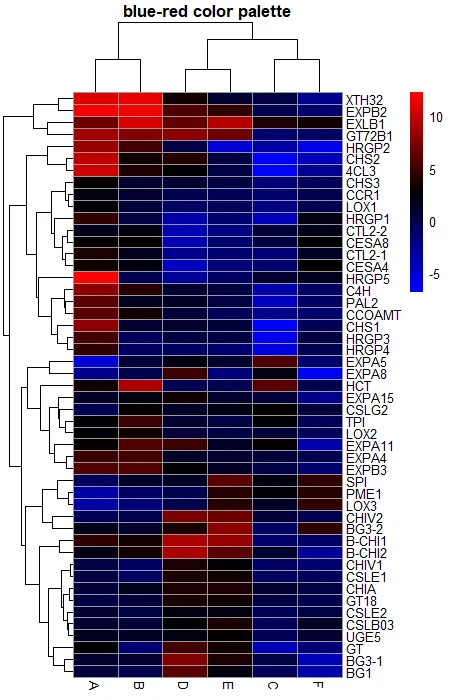You can add annotations to the row and columns to enhance the informative visualization for the heatmap. For example, you can add an annotation to a group of genes involved in the same biological pathway or you can add an annotation to the samples based on their conditions.

Here, I will add an annotation to genes (row) and samples (column) based on their gene functions and conditions.

``````# create a data frame for column annotation
smaple_group <- data.frame(sample = rep(c("infected", "control"), c(3, 3)))
row.names(smaple_group) <- colnames(df_mat)
# view data frame
smaple_group
sample
A infected
B infected
C infected
D  control
E  control
F  control

# create a data frame for row annotation
# view data frame
function.
B-CHI1 antifungal
CTL2-1 antifungal
B-CHI2 antifungal
CTL2-2 antifungal
CHIV1  antifungal
CHIA   antifungal

# heatmap with annotations
pheatmap(df_mat, annotation_row = gene_functions, annotation_col = smaple_group,
main = "heatmap with annotations")
``````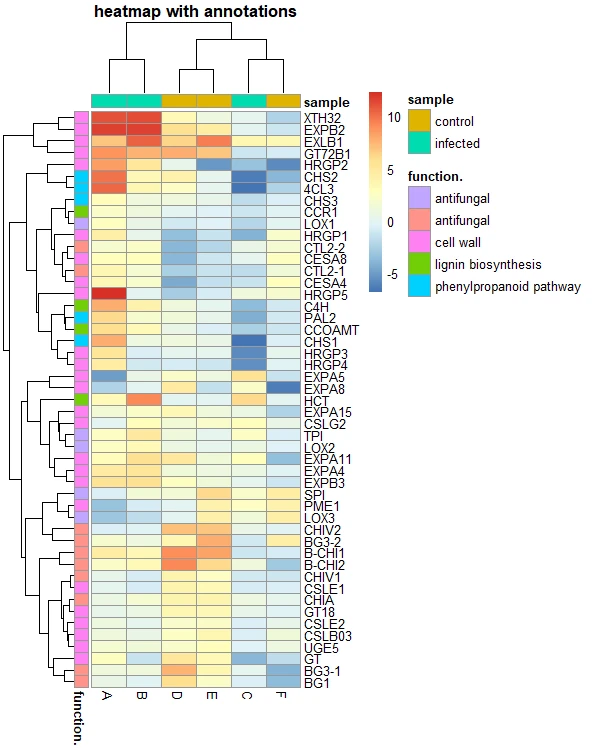### Split heatmap clusters

pheatmap provides parameters `cutree_rows` and `cutree_cols` to split the rows and columns based on the number of cluster. If you can visually see distinct clusters, you can provided that numbers to these paramaters. For example, for columns I can see three distinct clusters.

``````# heatmap with cluster break
pheatmap(df_mat, cutree_rows = 5, cutree_cols = 3, main = "heatmap with clusterized output")
``````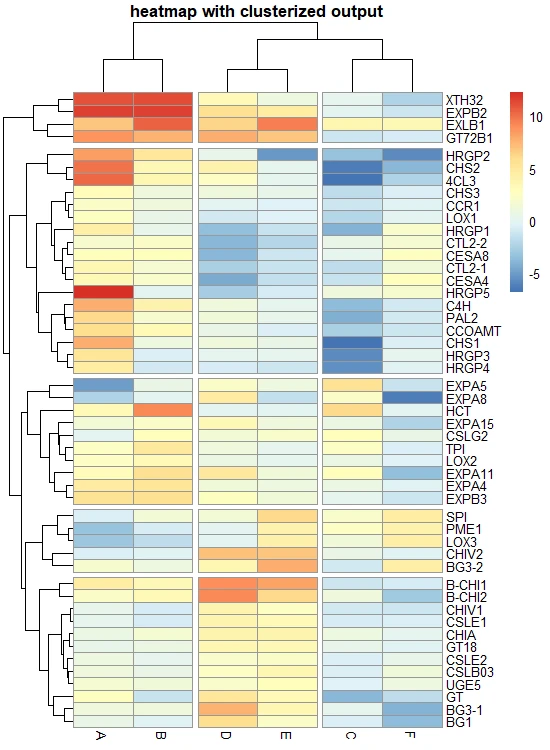Get the heatmap data belonging to each row cluster,

``````# get data that belong to row clusters
hm <- pheatmap(df_mat)
df_row_cluster = data.frame(cluster = cutree(hm\$tree_row, k = 5))
# view the genes clsuters
cluster
B-CHI1       1
CTL2-1       2
B-CHI2       1
CTL2-2       2
CHIV1        1
CHIA         1
``````

Get the heatmap data belonging to each column cluster,

``````# get data that belong to column clusters
hm <- pheatmap(df_mat)
df_col_cluster = data.frame(cluster = cutree(hm\$tree_col, k = 3))
# view the genes clsuters
cluster
A       1
B       1
C       2
D       3
E       3
F       2
``````

### Add gaps in the heatmap

In pheatmap, you can add gaps in heatmap between specific columns or rows to separate the heatmap. For example, you can add gaps to differentiate the heatmaps by the functional significance of the genes.

``````# add gap between 2-3 and 4-5 columns
pheatmap(df_mat, gaps_col = c(2, 4), gaps_row = c(5), cluster_cols = FALSE, cluster_row = FALSE,
border_color = FALSE, main = "heatmap with row and column gap")
``````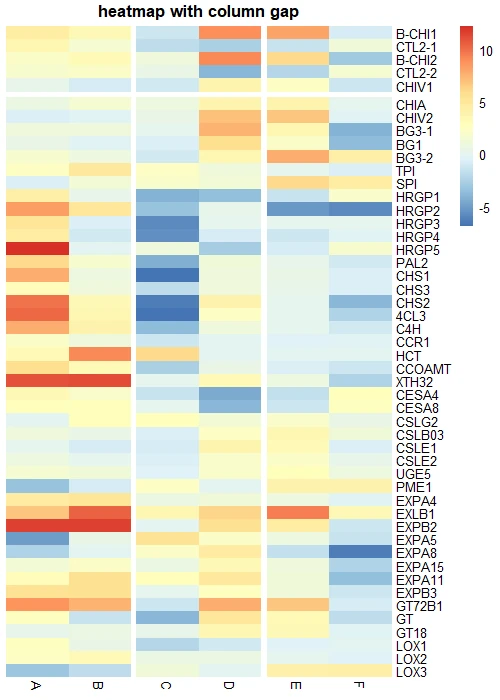Tip: If you would like to enable gaps in heatmaps, you must off row and column clustering

### Other parameters

In addition to the above function, you can also control other aesthetic parameters to control the fonts, border,

``````# heatmap with annotations
pheatmap(df_mat,
border_color = FALSE,      # no border to cell
fontsize_row = 5,          # row label font size
fontsize_col = 5,          # column label font size
angle_col = 90,             # angle for column labels
width = 5,                 # plot width in inches
height = 7,                # plot height in inches
na_col = "black",           # color of the cell with NA values
legend = FALSE,            # to draw legend or not (TRUE/FALSE)
filename = "heatmap.png"   # file name with path to save the heatmap
)
``````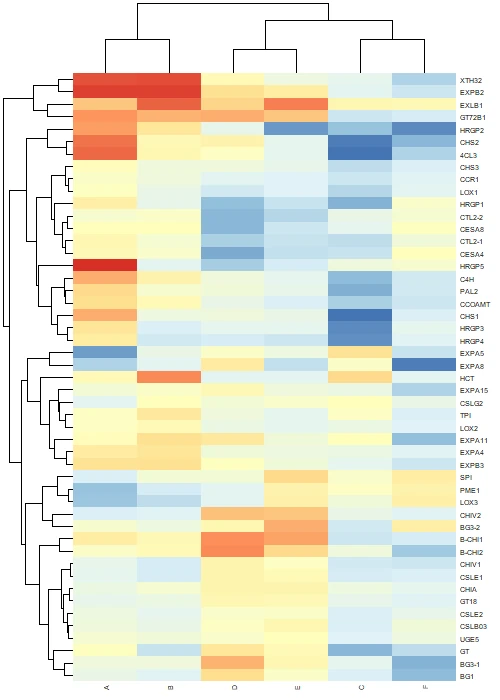Tip: If you save an image using the `filename` parameter, use `dev.off()` to close the plotting device